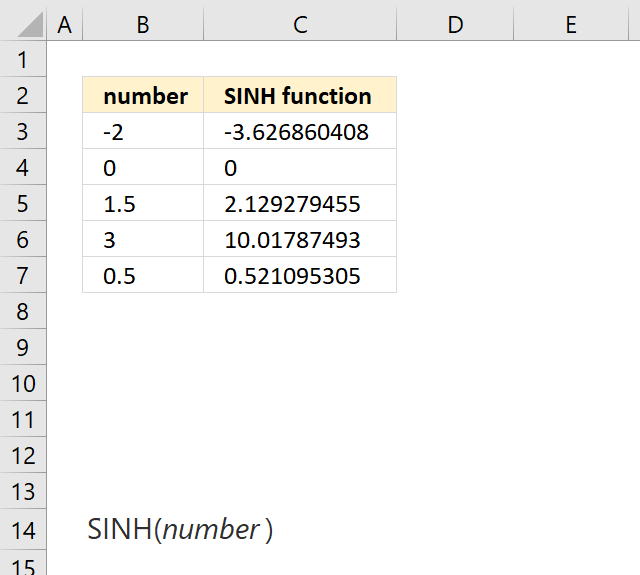Author: Oscar Cronquist Article last updated on May 04, 2018The SINH function calculates the hyperbolic sine of a number.

Formula in cell C3:

=SINH(B3)

SINH(number)

### Arguments

 number Required. Any real number.

### Comments

SINH(n) = (EXP(n)-EXP(-n))/2

How to use the EXP function

Returns e raised to the power of a number, e equals 2.71828182845904. Example, e^2 equals 7.389056099 Formula in cell C3: […]

SINH(n) = TANH(n)*COSH(n)